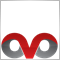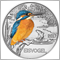# Shortcut to comment out code?17

Am starting to experiment with EAs and custom indicators and would like to know if there is a fast way to 'comment-out' (//) in one go code that is selected in MetaEditor. Figure there must be, but have been unable to find the solution so far.

Thanks in advance for any help.17213

marktheplant:

Am starting to experiment with EAs and custom indicators and would like to know if there is a fast way to 'comment-out' (//) in one go code that is selected in MetaEditor. Figure there must be, but have been unable to find the solution so far.

Thanks in advance for any help.

You mean a block of code ? comments

```      if (total == 1) OrderSelect(0,SELECT_BY_POS);
if (total == 1 && OrderType() == OP_SELL && Ask < Preis) {
Print ("3.+4.+1. aktiviert");
if (aktiv_2 == false) k++;

if (OrdersTotal() == 2) {
aktiv_4 = true;
aktiv_2 = false;
aktiv_RiskHedge = false;
k_multiplier = false;
if (aktiv_72 == true) {
OrderSelect(0,SELECT_BY_POS);
if (OrderType() == OP_SELL) OrderModify(OrderTicket(),0,StopLoss,0,0,CLR_NONE);
}
*/                                                                                                               // block ends here . . .
aktiv_72 = false;
Print ("k: ", k);
}
}```2895

I think he asks if the native MQL editor enables keyboard shortcuts for a line or block comments.

The answer is no, as far as I remember.17

Thanks RaptorUK and Ovo. Yes, was wondering whether there was a keyboard shortcut but your solution RaptorUK is just as good! Ta124

Comment: Ctrl + '

Uncomment: Ctrl + ;

However, "Ctrl + ;" doesn't work on my Mac installation. Does anybody know which is the Mac-shortcut for uncommenting?

Thanks!